##### Chemistry Workbook For Dummies with Online Practice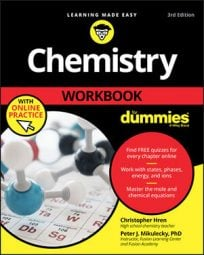Chemists have to be concerned with just how completely their reactants react to form products. To compare the amount of product obtained from a reaction with the amount that should have been obtained, they use percent yield. You determine percent yield of a chemical reaction with the following formula: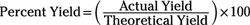That's nice and all, but what is an actual yield, and what is a theoretical yield? An actual yield is, well, the amount of product actually produced by the reaction in a lab or as told to you in the chemistry problem. A theoretical yield is the amount of product that could've been produced had everything gone perfectly, as described by theory if every single atom of reactants worked together perfectly. The theoretical yield is what you calculate when you do a calculation on paper or before you do a reaction in a lab.

The actual yield will always be less than the theoretical yield because no chemical reaction ever reaches 100 percent completion. In a lab setting, there's always some amount of error, whether it's big or small.

Try an example: Calculate the percent yield of sodium sulfate when 32.18 g of sulfuric acid reacts with excess sodium hydroxide to produce 37.91 g of sodium sulfate.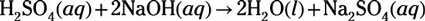First, note that the question clearly states that sodium hydroxide is the excess reagent. You always can ignore a reactant if the problem says it's in excess. That's like a big "this-one-isn't-important" sign in the problem.

So sulfuric acid is the limiting reagent and is the reagent you should use to calculate the theoretical yield: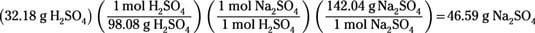Theory predicts that 46.59 g of sodium sulfate product is possible if the reaction proceeds perfectly and to completion. But the question states that the actual yield is only 37.91 g of sodium sulfate. With these two pieces of information, you can calculate the percent yield using the percent-yield formula: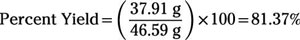So, you find that 81.37% is the percent yield.